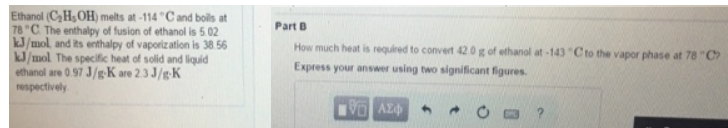# Problem: Ethanol (C2H3OH) melts at-114°Cand boils at 78°C. The enthalpy of fusion of ethanol is 5.02 kJ/mol and its enthalpy of vaporization is 38.56 kJ/mol. The specific heat of solid and liquid ethanol are 0 97 J/g • K are 2.3 J/g • K respectively.How much heat is required to convent 42.0 g of ethanol at -143 °C to the vapor phase at 78 °C? Express your answer using two significant figures.

###### FREE Expert Solution###### Problem Details

Ethanol (C2H3OH) melts at-114°Cand boils at 78°C. The enthalpy of fusion of ethanol is 5.02 kJ/mol and its enthalpy of vaporization is 38.56 kJ/mol. The specific heat of solid and liquid ethanol are 0 97 J/g • K are 2.3 J/g • K respectively.

How much heat is required to convent 42.0 g of ethanol at -143 °C to the vapor phase at 78 °C? Express your answer using two significant figures.